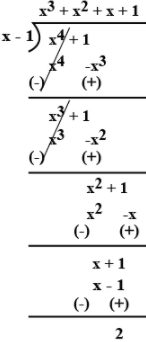# By actual division find the quotient and the remainder when (x4 + 1) is divided by (x - 1).

The division is as follows.$$\text { Quotient }=x^{3}+x^{2}+x+1 \\ \text { Remainder }=2$$(0)(0)